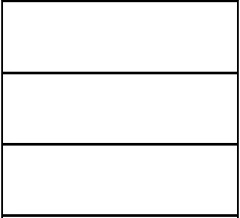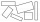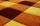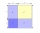# Two rectangles

I cut out two rectangles with 54 cm², 90 cm². Their sides are expressed in whole centimeters. If I put these rectangles together I get a rectangle with an area of 144 cm2. What dimensions can this large rectangle have? Write all options. Explain your calculation.

n =  6

### Step-by-step explanation:

The equations have the following integer solutions:
54=ab
90=bc

Number of solutions found: 6
##### a1=3, b1=18, c1=5a2=6, b2=9, c2=10a3=9, b3=6, c3=15a4=18, b4=3, c4=30a5=27, b5=2, c5=45a6=54, b6=1, c6=90

Calculated by our Diofant problems and integer equations.Did you find an error or inaccuracy? Feel free to write us. Thank you!Tips to related online calculators

#### You need to know the following knowledge to solve this word math problem:

We encourage you to watch this tutorial video on this math problem:

## Related math problems and questions:

• RectanglesHow many different rectangles with sides integers (in mm) have a circumference exactly 1000 cm? (a rectangle with sides of 50cm and 450cm is considered to be the same as a rectangle with sides of 450cm and 50cm)
• 144 stepsTo get around the garden we have to take 144 steps. 8 steps less in width than in length. One step is 5 decimetres long. What are the dimensions of the garden? What is its perimeter and its area?
• Rectangles - sidesOne side of the rectangle is 10 cm longer than a second. Shortens longer side by 6 cm and extends shorter by 14 cm increases the rectangle area by 130 cm2. What are the dimensions of the original rectangle?
• RemaindersIt is given a set of numbers { 170; 244; 299; 333; 351; 391; 423; 644 }. Divide this numbers by number 66 and determine set of remainders. As result write sum of this remainders.
• RectangleThe perimeter of the rectangle is 22 cm and content area 30 cm2. Determine its dimensions, if the length of the sides of the rectangle in centimeters is expressed by integers.
• Mr. ZucchiniMr. Zucchini had a rectangular garden whose perimeter is 28 meters. The garden's content area filled just four square beds, whose dimensions in meters are expressed in whole numbers. Determine what size could have a garden. Find all the possibilities and
• Do you solve this?Determine area S of rectangle and length of its sides if its perimeter is 102 cm.
• MartinaMartina is solving the equation 4x - 11 = 2x + 391. Here are the first steps of her solution. 4x - 11 = 2x + 391 2x - 11 = 391 2x = 402 What did Martina do to get 2x - 11 = 391?
• HarryHarry Thomson bought a large land in the shape of a rectangle with a circumference of 90 meters. He divided it into three rectangular plots. The shorter side has all three plots of equal length, their longer sides are three consecutive natural numbers. Fi
• TrianglesFind out whether given sizes of the angles can be interior angles of a triangle: a) 23°10',84°30',72°20' b) 90°,41°33',48°37' c) 14°51',90°,75°49' d) 58°58',59°59',60°3'
• RectanglesVladimir likes to draw rectangles. Yesterday he created all rectangles that had sides in centimeters and a circumference of 18 cm. How many rectangles of different dimensions have been drawn?
• Three rectanglesSome wire is used to make 3 rectangles: A, B, and C. Rectangle B's dimensions are 3/5 cm larger than Rectangle A's dimensions, and Rectangle C's dimensions are again 3/5 cm larger than Rectangle B's dimensions. Rectangle A is 2 cm by 3 1/5 cm. What is the
• RectanglesHow many different rectangles can be made from 60 square tiles of 1 m square? Find the dimensions of these rectangles.
• The carpetHow many meters of carpet 90 cm wide need to cover floor room which has a rectangular shape with a lengths 4.8 m and 2.4 m if the number of pieces on the carpet is needed to be lowest?
• Two rectangles 2A square of area 36 cm2 is cut out to make two rectangles. A and B The area of area A to area B is 2 : 1 Find the dimensions of rectangles A and B.
• Insulate houseThe property owner wants to insulate his house. The house has these dimensions 12, and 12 m is 15 m high. The windows have 6 with dimensions 170 and 150 cm. Entrance doors are 250 and 170 cm in size. How many square meters of polystyrene does he need?
• Rectangle diagonalsIt is given a rectangle with an area of 24 cm2 a circumference of 20 cm. The length of one side is 2 cm larger than the length of the second side. Calculate the length of the diagonal. Length and width are yet expressed in natural numbers.